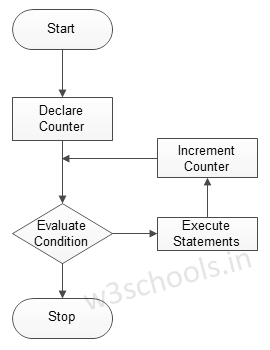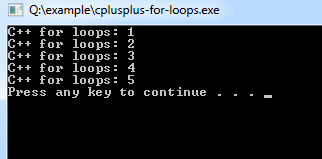## C++ Programming Tutorial Index

C++ `for` loop is similar to the `while` loop; It continues to execute a block of code until the predefined condition is met. This is an entry-controlled loop. This tutorial will teach you how to use the `for` loop in C++.

The basic format of the `for` loop statement is:

Syntax:

``````for ( init; condition; increment )
{
statement(s);
}``````

Figure - Flowchart of the `for` loop:Example C++ program to demonstrate the `for` loop:

Example:

``````#include <iostream>
using namespace std;

int main ()
{
/* local variable Initialization */
int n = 1, times=5;

/* for loops execution */
for( n = 1; n <= times; n = n + 1 )
{
cout << "C++ for loops: " << n <<endl;
}
return 0;
}``````

Program Output: